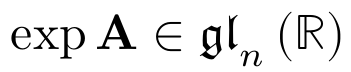# math-variant explainer

This document proposes new values for the text-transform properties in order to map alphanumeric text to equivalent Mathematical Alphanumeric Symbols. It will help implementers of MathML (or more generally of tools to generate or display math content) by taking care of inheritance, character mapping and miscellaneous other use cases. See math-variant tables for detailed per-character conversion. For further discussions on this proposal see math-variant comments.

## Examples

• <span style="text-transform: math-fraktur">A</span> would render the same as <span>𝔄</span> or <span>&#x1D504;</span> (U+1D504 MATHEMATICAL FRAKTUR CAPITAL A).

• The LaTeX formula \exp{\mathbf{A}} \in _n\left(\mathbb R\right)} is written with the exponential function using “normal” variant, the matrix A using “bold” variant, the Lie algebra using “fraktur” variant, the integer n using “italic” variant and the set of numbers R using “double-struck” variant. The \math* commands could just be mapped to equivalent HTML nodes, styled with the text-transform property.• A simple math formula like <span style="text-transform: math-italic" style>x + y</span> would have x, y in italic but not operator + (contrary to font-style: italic).

• <span style="font-family: STIX Math; text-transform: math-bold">F</span> would use the glyph for U+1D46D MATHEMATICAL BOLD ITALIC CAPITAL F contained in the STIX Math font. It would not try to look into the (non-existent) STIX Math bold font or emulate bold style from the F glyph of the STIX Math font. However, if STIX Math font is not available and none of the fonts used actually have a glyph for U+1D46D it may use this kind of fallback behavior, as it is done for font-weight: bold.

• Polyfills can emulate MathML behavior of <mstyle mathvariant="double-struck">...</mstyle> via mstyle[mathvariant="double-struck"] { text-transform: math-double-struck; }. More generally, native implementations could directly map the MathML mathvariant attribute to the CSS text-transform property.

## Rationale

• Mathematical formulae rely on mathematical alphanumeric symbols to provide specific meanings. For example, the Planck constant is denoted by an italic h, vectors in physics are often written as bold variables, set of numbers are represented by double-struck and Lie algebras by fraktur letters. One can rely on properties such as font-style or font-weight to render some of these symbols from basic alphanumeric characters but this is not possible for others like fraktur, double-struck, calligraphic, etc

• Unicode contains characters to describe this semantic information but they are difficult to typeset with standard keyboard. It might also be complicated for basic math parsers or renderers to properly inherit mathvariant and perform transformations from the original characters. For example in LaTeX, $x + \mathfrak{\mathbb{\aleph+N} \sin \frac{2}{\alpha}}$ makes x italic, N double-struck, 2 fraktur and all the other characters are unchanged. Hence a CSS property similar to text-transform to easily perform transformation and inheritance would be helpful.

• Most OpenType math fonts have glyphs to represent these characters but don’t have associated italic/bold/bold-italic fonts. For generic fonts, the situation is actually the opposite: italic/bold/bold-italic fonts are available but not the corresponding mathematical alphanumeric symbols. It is tedious for math content generators or renderers to handle these different cases and decide some reasonable fallback, so a standardized approach is needed.

• One important math typesetting convention is that single-character identifiers are italic by default. Again, since OpenType math fonts have italic mathematical alphanumeric symbols but no associated italic fonts, using font-style: italic does not provide the same visual rendering as the equivalent mathematical alphanumeric symbols.

• MathML defines a mathvariant attribute to facilitate performing these mathvariant transformations from the basic alphanumeric characters, some kind of inheritance (e.g. like \mathfrak above), automatic italic on single-character <mi> elements. Some fallback for things like italic or bold are also suggested. Mapping the mathvariant attribute to some CSS property would allow an implementation that is consistent with CSS inheritance or text-transform.

## Status in Web Engines

• text-transform is implemented in all engines.
• The mathvariant attribute is implemented in Gecko and Stylo via an internal CSS property. It could be aligned with this specification with some refactoring and adjustments.
• The mathvariant attribute is partially implemented in WebKit using some custom CSS-like inheritance and changing text rendering in token elements but only works for single-character identifier (e.g. does not handle <mi mathvariant="fraktur">sl</mi>). Relying on a CSS property would allow a cleaner and more complete implementation.
• There are MathML tests for mathvariant that could easily be converted to test the new CSS text-transform values. Gecko has layout tests for other edge cases described in this specification.

## Proposal

### New CSS text-transform values

See https://mathml-refresh.github.io/mathml-core/#new-text-transform-values

### Native implementation of the mathvariant attribute

See https://mathml-refresh.github.io/mathml-core/#attribute-mathvariant